# Argument Handling in PythonArgument handling gives user the chance to interact with the script and use it for different tasks. Two ways to do this in Python are with ARGV and optparse. The optparse module offers more options for argument handling, but we will use the sys module for our simple example to demonstrate argument handling in Python programming language.

So far we have created simple scripts with a static nature, now it is time to create Python scripts that interact with the user. We need the sys module to use ARGV structure which is a list containing the name of the program (the name of the script in our case) and all arguments passed by the user in the command-line. How does the user know what arguments to pass in the command-line? We will use the sys.exit() function to inform the user about the program usage if the user does not have any information about its usage. We will build a simple calculator that finds the sum of two numbers passed by the user in the command-line. Open a new terminal and use your favorite text editor to create a Python file with the name ‘calculator.py’ like shown in Figure 1.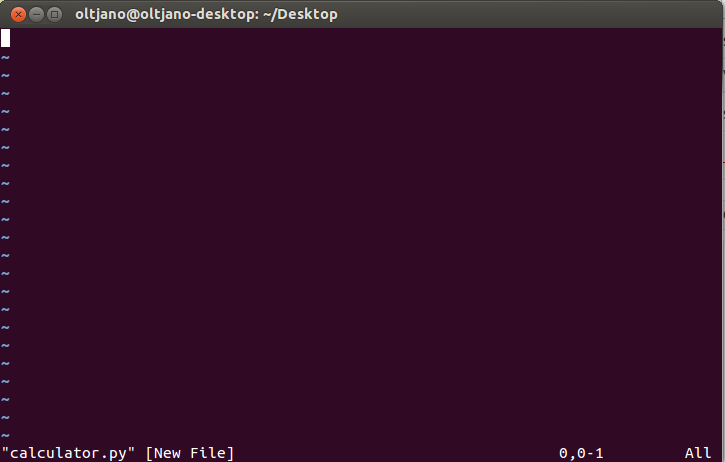Figure 1

After adding the shebang line it is the time to import necessary modules for our program.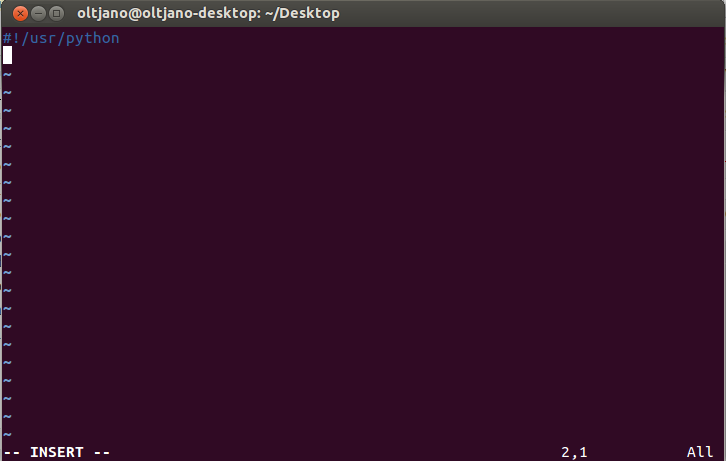Figure 2

The sys module will help us to handle user arguments passed in the command-line, so we import this important module.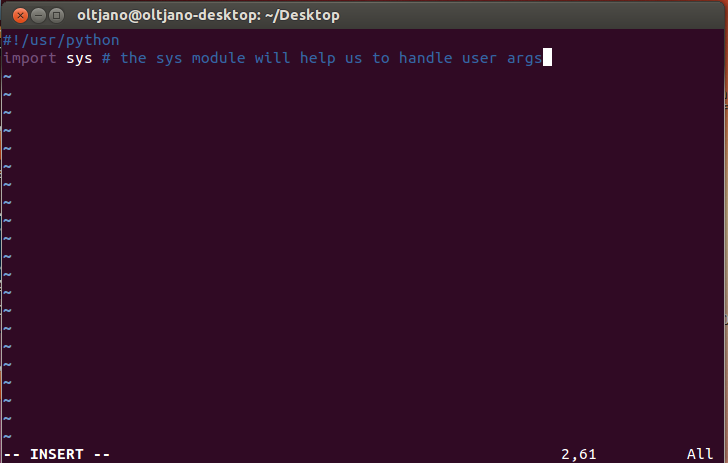Figure 3

Our program will calculate the sum of two numbers so the sys.argv list will contain three elements, the name of the program and the two numbers that will be passed by the user in the command-line. We will use an if conditional to check if the length of the sys.argv list is equal to three and if not, we will tell the user how to use the program.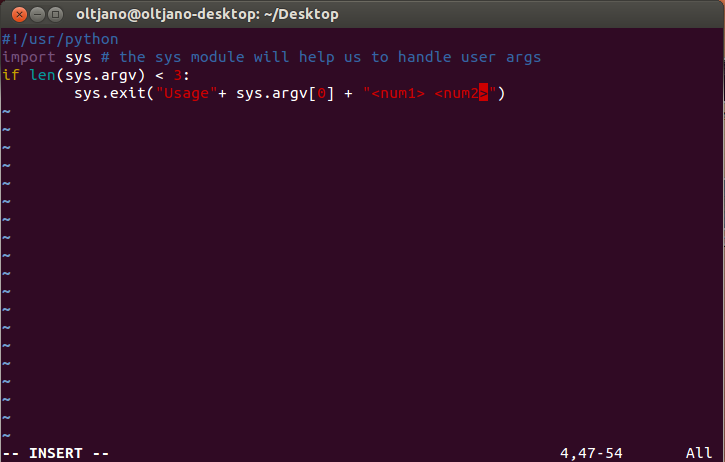Figure 4

We use the sys.exit() function to exit the system and inform the user about the program usage. If we run the program now without any argument the output will be the same with the output in Figure 5.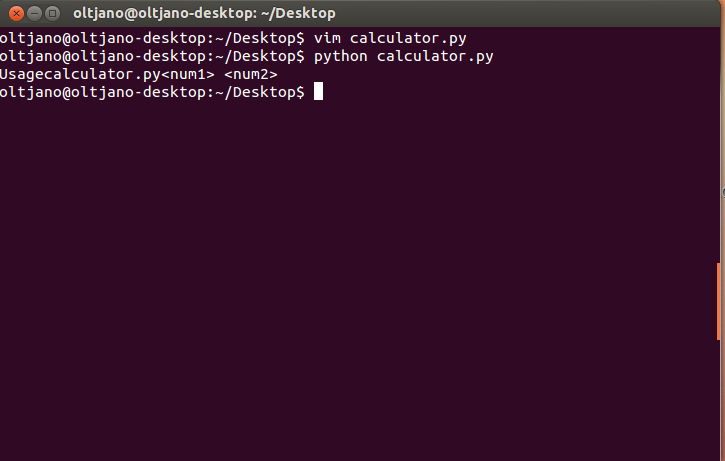Figure 5

Until now, our program works pretty well. We will use sys.argv to get the user input from the command-line. The first number is sys.argv and the second number is sys.argv. The sys.argv element of the list is the name of the program, in our case it is calculator.py. We use two variables, num1 and num2 like shown in Figure 6.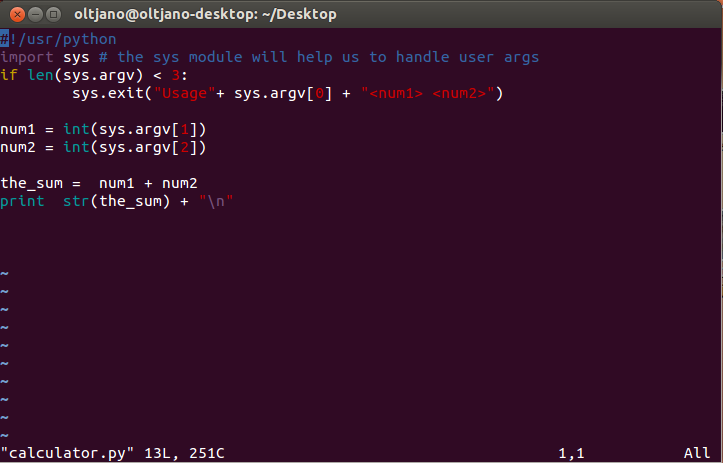Figure 6Figure 7

After linking numbers to variables the heavy work is done, we add the two numbers together and print the sum on the screen. Figure 8 shows how our programs works.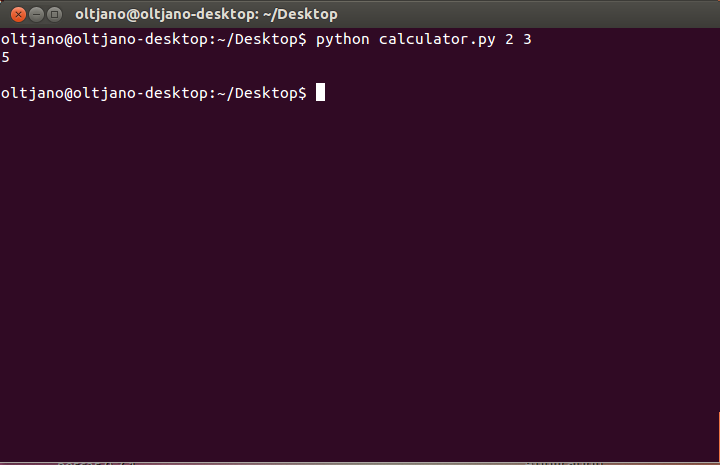Figure 8

I hope you find very helpful in learning Argument Handling in Python this simple example.